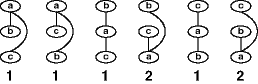Guide to Constraint Programming © Roman Barták, 1998 Contents Prev Up Next

# Reducing Search

[background] [cycle-cutset] [MACE] [heuristics]

In the previous section on Value and Variable Ordering we presented orderings which can noticeably improve the efficiency of backtrack search. The question is: Does there exist any variable ordering which can eliminate the need for backtracking? Before answering this question we define some terms.

###Background

An ordered constraint graph is a constraint graph whose vertices have been ordered linearly. The width of the vertex in an ordered constraint graph is the number of constraint arcs that lead from the vertex to the previous vertices (in the linear order). The width of the ordered constraint graph is the maximum width of any of its vertices and the width of the constraint graph is the minimum width of all the orderings of that graph. In general the width of a constraint graph depends upon its structure.

 Example:constraint graphdifferent ordered constraint graphs widths of the ordered constraint graphs The width of the constraint graph is 1.

Theorem: If a constraint graph is strongly K-consistent, and K>w where w is the width of the constraint graph, then there exists a search order that is backtrack free.

The proof of the above theorem is straightforward. There exists an ordering of the graph such that the number of constraint arcs leading from any vertex of the graph to the previous vertices is at most w. Now if the variables are instantiated using this ordering, then whenever a new variable is instantiated, a value for this variable is consistent with all the previous assignments because: (i) this value is to be consistent with the assignments of at most w other variables, and (ii) the graph is strongly (w+1)-consistent.

Interestingly, all tree structured constrained graphs have width 1, so it is possible to find at least one ordering that can be used to solve the constraint graph without backtracking provided that the constraint graph is arc consistent.

###Cycle-Cutset

According to the above theorem, acyclic constraint graphs are globally consistent iff they are arc consistent. So, if we first instantiate consistently the nodes/variables which "cut" all the cycles in a graph (the set of such nodes is called cycle cutset) and make the graph arc consistent then it is possible to find ordering of rest variables that eliminate the need for backtracking. This observation makes the basis of the cycle cutset algorithm that solves the cycle cutset independently and then extends this partial solution to a complete solution in a backtrack-free manner using the variable ordering described in the above section.

#### Algorithm Cycle-Cutset

```1) partition the variables into two sets:
a cycle-cutset C, and T, the complement of C;
2) find a(nother) solution to the the problem with variables C only
if no solution can be found then return fail
3) enforce directed arc consistency from C to T
if any domain is empty then backtrack to 2
4) use backtrack-free search for extending the partial solution to a complete solution;```

The major problem with the cycle cutset method is its potential for thrashing, i.e., occurrence of backtracks in the step 3 of above algorithm.

###MACE (MAC Extended)

MACE is an extended version of the popular algorithm MAC that combines two ideas: instantiate less and propagate less. MACE instantiates only a subset of the CSP variables while maintaining only a partial arc consistent state of the constraint network. The gain in efficiency is twofold. Instantiating a smaller number of variables aims at reducing the number of backtracks (and, accordingly, the number of constraint-checks, nodes visited, values deleted, etc.).

MACE uses the idea of cycle cutset of a constraint graph while removes its major drawback, thrashing, by maintaining arc consistency.

#### Algorithm MACE

```1) enforce arc consistency (if it is not possible then return fail)
2) partition the variables into two sets:
a cycle-cutset C and the set of variables which are not involved in any cycle U
disconnect U from the graph
3) while C#0 do
3a) set value to a variable in C
3b) enforce arc consistency
if failed then backtrack to 3a
3c) disconnect singleton variables (add them to U)
3d) disconnect "cycle-free" variables (add them to U)
4) reconnect variables in U
enforce directed arc consistency from C to U
5) conduct backtrack-free search for a complete solution```

###Heuristics

MACE and CC need an algorithm to find a cycle-cut. There is no known polynomial algorithm for finding the minimum cycle-cutset. Fortunately, there are several heuristics which yield a good cycle-cutset at a reasonable cost.
1. The simplest heuristic sorts first the variable in decreasing order of their degree. Then, starting with the variable with the highest degree, as long as the graph still has cycles, add the variable to the cycle-cutset and remove it, together with all the edges involving it, from the graph.
2. A smaller cutset can be obtained if, before adding a variable to the cutset, we check whether it is part of any cycle or not.
3. The third heuristic determines dynamically the number of cycles in which each variable is involved and adds to the cutset at each step the variable participating in the most cycles.

Visibly, second and third heuristic yields smaller cutsets than the first heuristic. Based on real-life observations, the third heuristic yields slightly smaller cutsets which translate sometimes into small gains in efficiency, but no major improvement upon the second heuristic. In case of large problems probably the second heuristic is the best choice, because of its lower cost.

[background] [cycle-cutset] [MACE] [heuristics]

 Contents Prev Up Next Designed and maintained by Roman Barták